Research activities Rational Thermodynamics Physical chemistry is the

• Slides: 19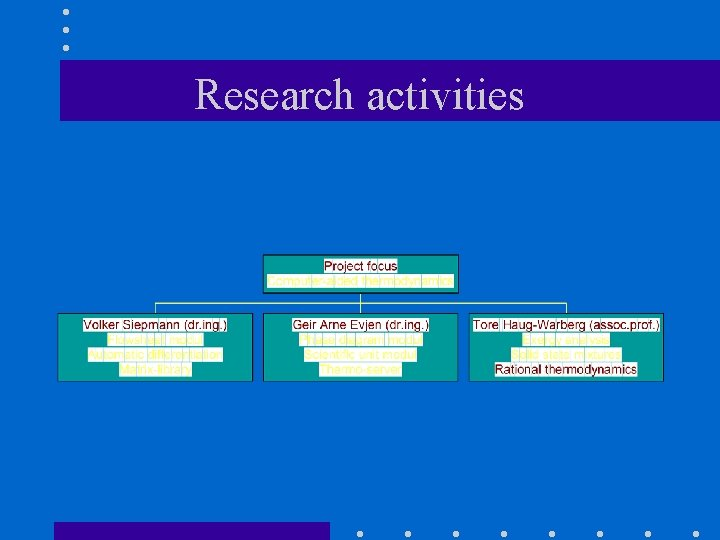Research activitiesRational Thermodynamics • Physical chemistry is the ancestor of chemical engineering thermodynamics: => Inheritance of definitions originally introduced to please the experimentator. => Theory is adequate for hand calculations, but quite disadvantageous for general computer programming.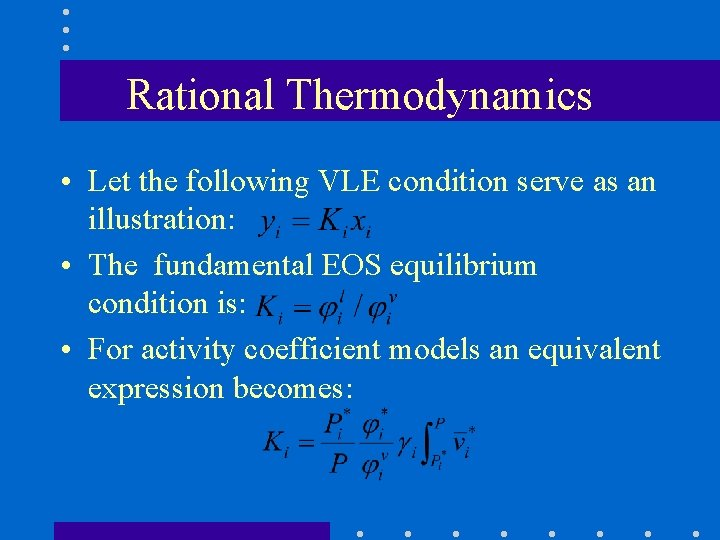Rational Thermodynamics • Let the following VLE condition serve as an illustration: • The fundamental EOS equilibrium condition is: • For activity coefficient models an equivalent expression becomes:Rational Thermodynamics • For supercritical components a Henry reference state will be more appropriate: • There is now a bewildering number of symbols & indices:Rational Thermodynamics • Complex notation => model errors may occur at two different levels: 1) Inconsistent approximations made at the implementation level (semantic bugs). 2) Inconsistent use of model features in the user interface (syntactic bugs).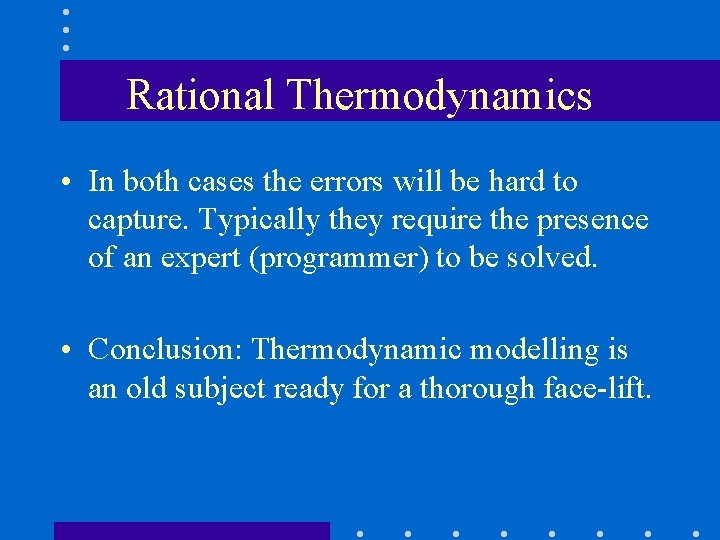Rational Thermodynamics • In both cases the errors will be hard to capture. Typically they require the presence of an expert (programmer) to be solved. • Conclusion: Thermodynamic modelling is an old subject ready for a thorough face-lift.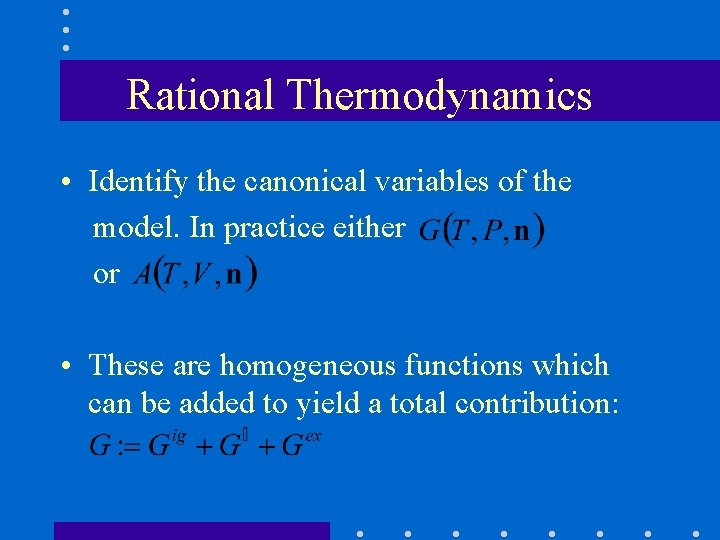Rational Thermodynamics • Identify the canonical variables of the model. In practice either or • These are homogeneous functions which can be added to yield a total contribution:Rational Thermodynamics • The standard state contribution can be split into new (sub)contributions following the same rules: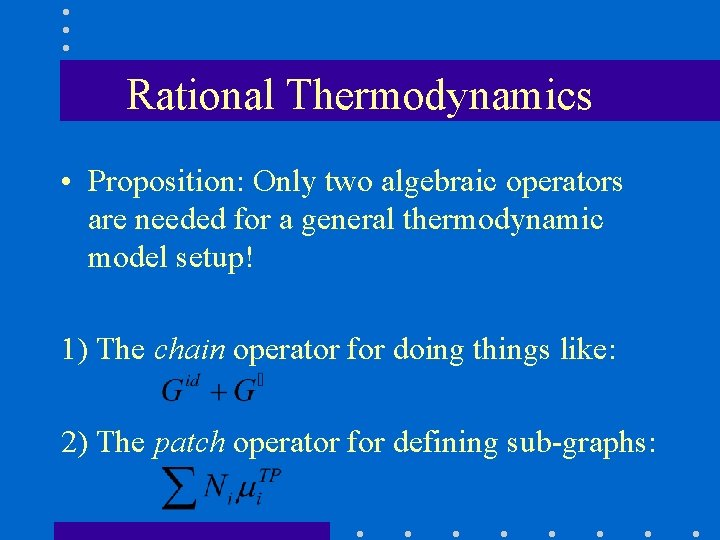Rational Thermodynamics • Proposition: Only two algebraic operators are needed for a general thermodynamic model setup! 1) The chain operator for doing things like: 2) The patch operator for defining sub-graphs: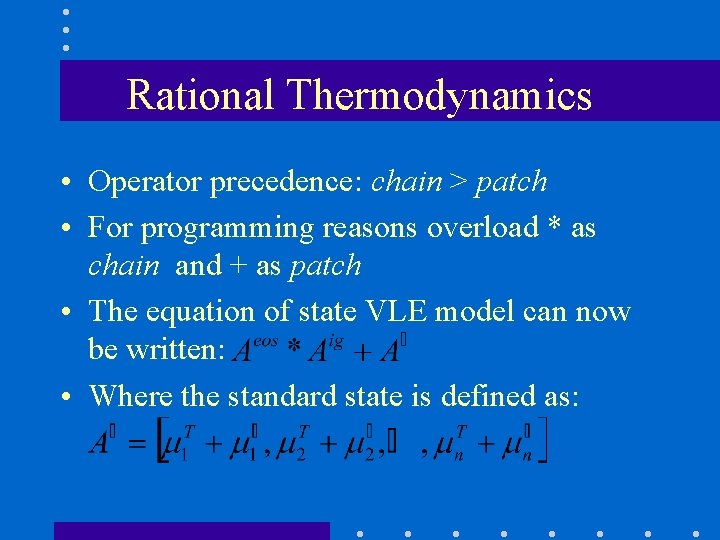Rational Thermodynamics • Operator precedence: chain > patch • For programming reasons overload * as chain and + as patch • The equation of state VLE model can now be written: • Where the standard state is defined as: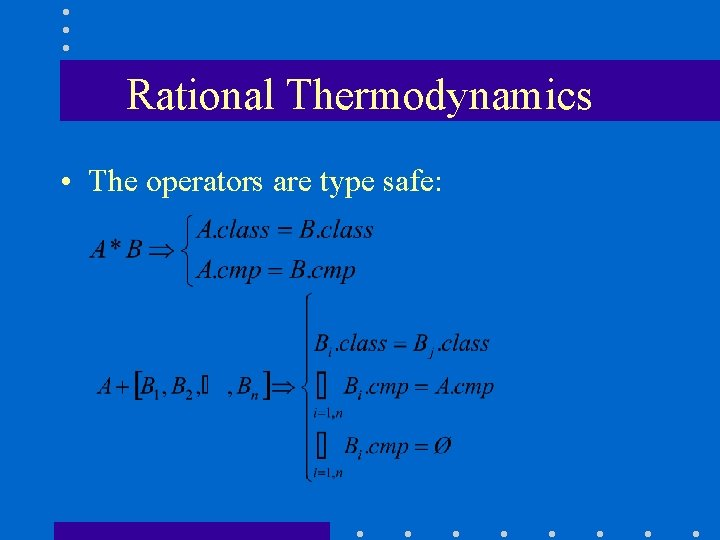Rational Thermodynamics • The operators are type safe: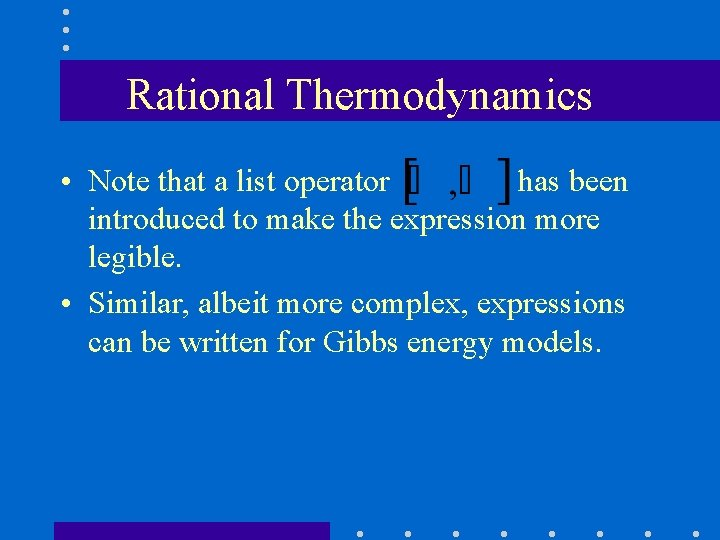Rational Thermodynamics • Note that a list operator has been introduced to make the expression more legible. • Similar, albeit more complex, expressions can be written for Gibbs energy models.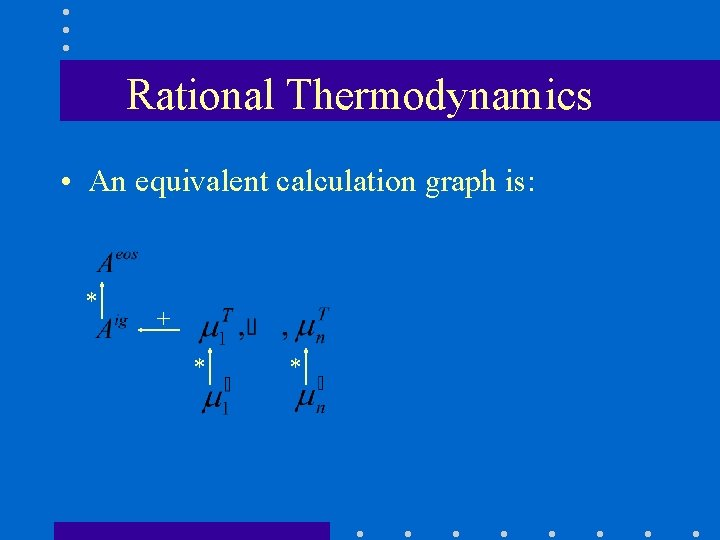Rational Thermodynamics • An equivalent calculation graph is: * + * *Rational Thermodynamics • Example code (in Ruby for a change): mix = [”H 2 O”, ”CH 3 OH”, ”C 2 H 5 OH”] A 0 = Mu. T. new(mix) * [[: cp. Dippr, ”ig”] + Mu. new(mix) * [: Ho. So, ”ig”]] A = Helmholtz. new(mix) * [: cubic. EOS, ”fl”] * [: ideal. Gas, ”ig”] + A 0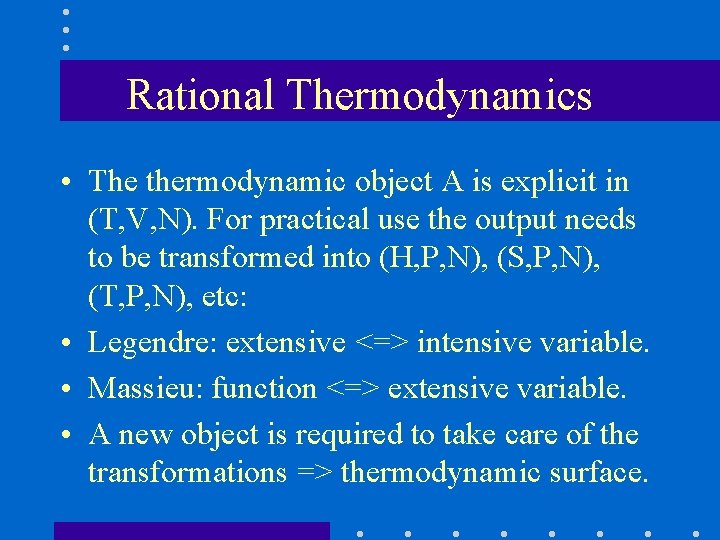Rational Thermodynamics • The thermodynamic object A is explicit in (T, V, N). For practical use the output needs to be transformed into (H, P, N), (S, P, N), (T, P, N), etc: • Legendre: extensive <=> intensive variable. • Massieu: function <=> extensive variable. • A new object is required to take care of the transformations => thermodynamic surface.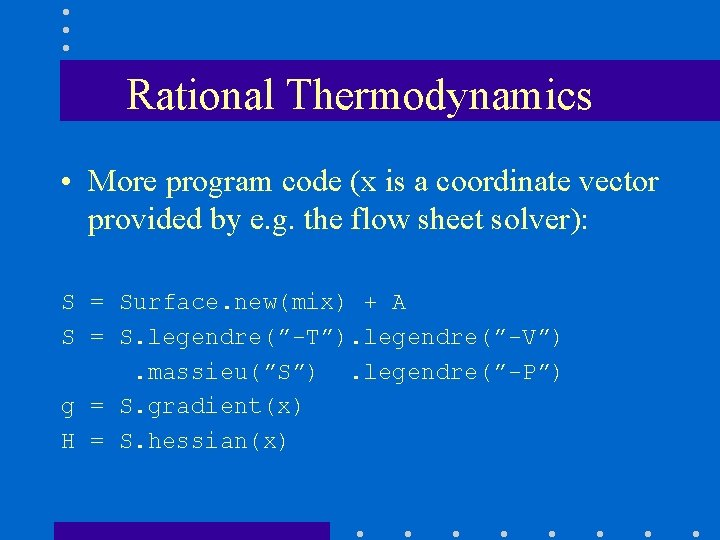Rational Thermodynamics • More program code (x is a coordinate vector provided by e. g. the flow sheet solver): S = Surface. new(mix) + A S = S. legendre(”-T”). legendre(”-V”). massieu(”S”). legendre(”-P”) g = S. gradient(x) H = S. hessian(x)Rational Thermodynamics • Thermo-objects are made by operators but modified by methods => clean interface. • Use of operators => thermodynamic frameworks can be broken down into small, manageable, sequences. • Model description is not tied to any particular implementation => easy to export, exchange and update model info.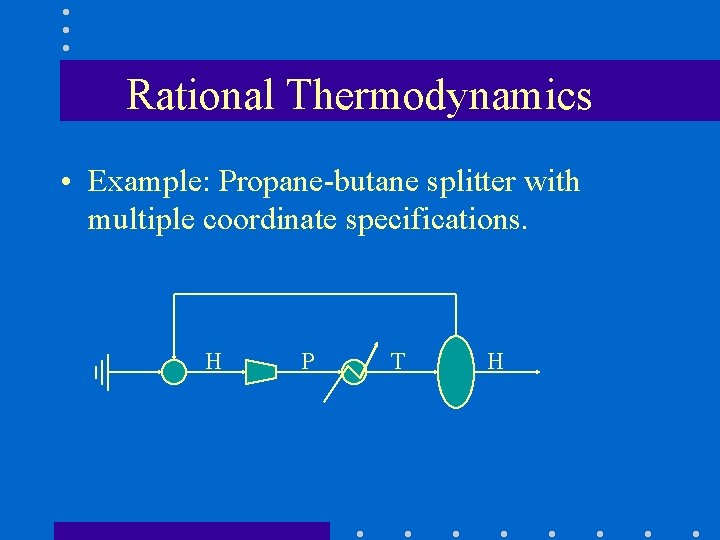Rational Thermodynamics • Example: Propane-butane splitter with multiple coordinate specifications. H P T H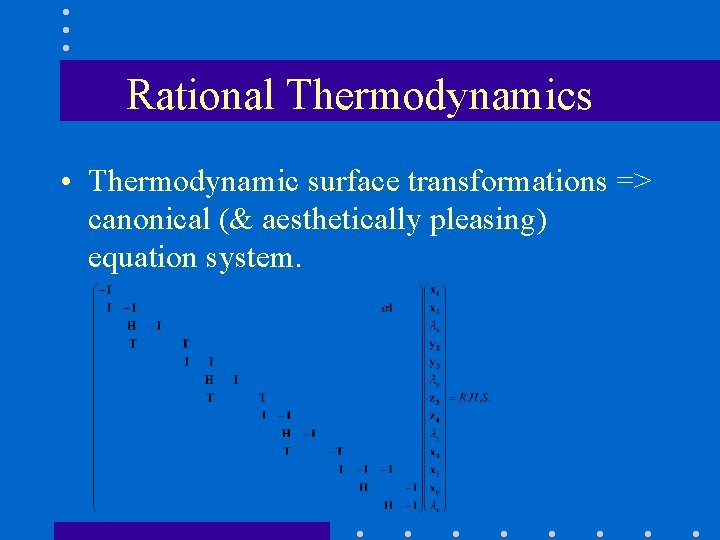Rational Thermodynamics • Thermodynamic surface transformations => canonical (& aesthetically pleasing) equation system.